# Čech cohomology

(diff) ← Older revision | Latest revision (diff) | Newer revision → (diff)

Aleksandrov–Čech cohomology, spectral cohomology

The direct limit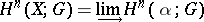of the cohomology groups with coefficients in an Abelian groupof the nerves of all open coveringsof a topological space. The cohomology group of a closed subsetcan be defined analogously, using the subsystem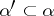of all such sets fromthat have non-empty intersection with. The limit of the groups of the pairdefines the cohomology groupof the pair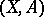. The cohomology sequence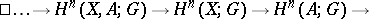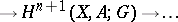of the pairis exact, being the limit of the exact cohomology sequences of the pairs of nerves of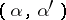.

Aleksandrov–Čech cohomology serves as a substitute for singular cohomology in general categories of topological spaces, and agrees with it whenever the applicability of the latter is not in doubt (specifically, in the case of homologically, locally connected spaces, in particular locally contractible spaces). It satisfies all Steenrod–Eilenberg axioms, and on the category of paracompact spaces it is uniquely determined by those axioms together with the following conditions: a)for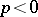; b) the cohomology group of a discrete union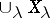is naturally isomorphic to the direct product of the cohomology groups of the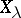; and c)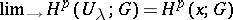for the system of all neighbourhoods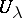of an arbitrary point. Aleksandrov–Čech cohomology is isomorphic to Alexander–Spanier cohomology. The latter can be defined with coefficients in a sheaf, and for paracompact spaces it is isomorphic to the cohomology defined in sheaf theory.

The approximability of spaces by polyhedra — by the nerves of closed coverings — was established by P.S. Aleksandrov (cf. ). For a particular case he has given the definition of an inverse limit of topological spaces, and, on the basis of the approximation, the definition of the Betti numbers of metrizable compacta. The homology groups of compacta are defined in terms of Vietoris cycles. L.S. Pontryagin  introduced direct and inverse spectra of groups, and applied these notions to the study of the homology groups of compacta. E. Čech began to consider nerves of finite open coverings of non-compact spaces and on this basis initiated the homology theory of arbitrary topological spaces. It turned out later that it is not justifiable to consider only finite open coverings (since this leads to the rather complicated homology of the Stone–Čech compactification). C.H. Dowker  demonstrated that it is fruitful to use arbitrary open coverings in the homology and cohomology theory of non-compact spaces.

How to Cite This Entry:
Čech cohomology. Encyclopedia of Mathematics. URL: http://encyclopediaofmath.org/index.php?title=%C4%8Cech_cohomology&oldid=17632
This article was adapted from an original article by E.G. Sklyarenko (originator), which appeared in Encyclopedia of Mathematics - ISBN 1402006098. See original article